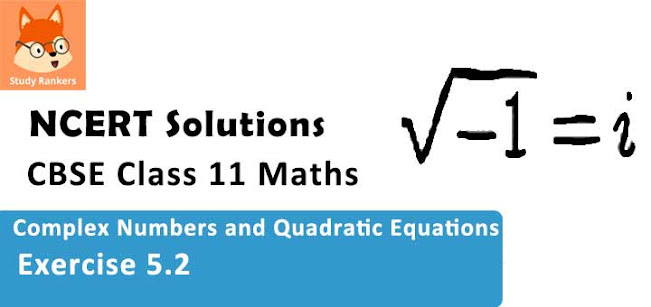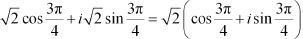# Class 11 Maths NCERT Solutions for Chapter 5 Complex Numbers and Quadratic Equations Exercise 5.2### Complex Numbers and Quadratic Equations Exercise 5.2 Solutions

1. Find the modulus and the argument of the complex number z = -1 - i√3

Solution

z = -1- i√3
Let r cos θ = -1 and r sin θ = -√3
On squaring and adding, we obtain
(r cos θ)2 + (r sin θ)2 = (-1)2 + (-√3)2
⇒ r2 (cos2 θ + sin2 θ) = 1 + 3
⇒  r2 = 4  [cos2 θ + sin2 θ = 1]
⇒ r = √4 = 2  [Conventionally, r > 0]
∴ Modulus = 2
∴ 2 cos θ = -1 and 2sin θ = - √3
⇒ cos θ = -1/2  and sin θ = -√3/2
Since both the values of sin θ and cos θ are negative and sin θ and cos θ are negative in III quadrant,
Argument = -(π - π/3) = -2π/3
Thus, the modulus and argument of the complex number -1 - √3 i are 2 and -2π/3 respectively.

2. Find the modulus and the argument of the complex number z = -√3 + i

Solution

z = -√3 + i
Let r cos θ = -√3 and r sin θ = 1
On squaring and adding, we obtain
r2 cos2 θ + r2 sin2 θ = (-√3)2 + 12
⇒ r2 = 3 + 1 = 4  [cos2 θ + sin2 θ = 1]
⇒ r = √4 = 2  [Conventionally, r > 0]
∴ Modulus = 2
∴ 2 cos θ = -√3 and 2sin θ = 1
⇒ cosθ = -√3/2 and sinθ = 1/2
∴ θ = π - π/6 = 5π/6  [As θ lies in the II quadrant]
Thus, the modulus and argument of the complex number -√3 + i are 2 and 5π/6 respectively.

3. Convert the given complex number in polar form : 1 - i

Solution

1 - i
Let r cos θ = 1 and r sin θ = -1
On squaring and adding, we obtain
r2 cos2 θ + r2 sin2 θ = 12 + (-1)2
⇒ r2 (cos2 θ + sin2 θ) = 1 + 1
⇒ r2 = 2
⇒ r = √2  [Conventionally, r > 0]
∴ √2 cos θ = 1 and √2 sin θ = -1
⇒ cos θ = 1/√2  and sinθ = -1/√2
∴ θ = -π/4  [As θ lies in the IV quadrant]
∴ 1 - i = r cos θ + i r sinθ = √2 cos (-π/4)  + i√2sin(-π/4) = √2[cos (-π/4) + i sin(-π/4) ] This is the required polar form.

4. Convert the given complex number in polar form : -1 +i

Solution

-1+ i
Let r cos θ = -1 and r sin θ = 1
On squaring and adding, we obtain
r2 cos2 θ + r2 sin2 θ = (-1)2 + 12
⇒ r2 (cos2 θ + sin2 θ) = 1 + 1
⇒ r2 = 2
⇒ r = √2  [Conventionally, r > 0]
∴ √2 cos θ = -1 and √2sin θ = 1
⇒ cos θ = -1/√2 and sinθ = 1/√2
∴ θ = π - π/4 = 3π/4  [As θ lies in the II quadrant]
It can be written,
∴ -1 + i = r cos θ + i r sinθThis is the required polar form.

5. Convert the given complex number in polar form : -1 - i .

Solution

-1-i
Let r cos θ = -1 and r sin θ = -1
On squaring and adding, we obtain
r2 cos2 θ + r2 sin2 θ = (-1)2  + (-1)2
⇒ r2 (cos2 θ + sin2 θ) = 1 + 1
⇒ r2 = 2
⇒ r = √2  [Conventionally, r > 0]
∴ √2 cos θ = -1 and √2sin θ = -1
⇒ cos θ = -1/√2 and sinθ = -1/√2
∴ θ = -(π - π/4) = -3π/4  [As θ lies in the III quadrant]
∴ -1-i = r cos θ + i r sin θ = √2 cos (-3π/4) + i√2 sin(-3π/4) = √2[cos(-3π/4) + i sin(-3π/4)]
This is the required polar form.

6. Convert the given complex number in polar form: -3

Solution

-3
Let r cos c = -3 and r sin θ = 0
On squaring and adding we obtain
r2 cos2 θ + r2 sin2 θ = (-3)2
⇒ r2 (cos2 θ + sin2 θ) = 9
⇒ r2 = 9
⇒ r = √9 = 3  [Conventionally, r > 0]
∴ 3 cosθ = -3 and 3 sinθ = 0
⇒ cos θ= -1 and sinθ = 0
∴ θ = π
∴ -3 = r cos θ + i r sin θ = 3 cos π + B sinπ = 3(cos π + isin π)
This is the required polar form.

7. Convert the given complex number in polar form : √3 + i

Solution

√3 + i
let r cos θ = √3 and r sin θ = 1
On squaring and adding, we obtain
r2 cos2 θ + r2 sin2 θ = (√3)2 + 12
⇒ r2 (cos2 θ + sin2 θ) = 3 + 1
⇒ r2 = 4
⇒ r = √4 = 2   [Conventionally, r > 0]
∴ 2 cosθ = √3 and 2sinθ = 1
⇒ cos θ = √3/2 and sinθ = 1/2
∴ θ = π/6  [As θ lies n the I quadrant]
∴ √3 + i = r cos θ + i r sinθ = 2 cos (π/6 ) + i 2 sin (π/6) = 2[cos(π/6) + i sin(π/6)]
This is the required polar form.

8. Convert the given complex number in polar form : i

Solution

i
Let r cosθ = 0 and r sin θ = 1
On squaring and adding, we obtain
r2 cos2 θ + r2 sin2 θ = 02 + 12
⇒ r2 (cos2 θ + sin2 θ) = 1
⇒ r2 = 1
⇒ r = √1 = 1  [Conventionally, r > 0]
∴ cosθ = 0 and sinθ = 1
∴ θ = π/2
∴ i = r cosθ + i r sin θ = cos (π/2) + i sin (π/2)
This is the required polar form.